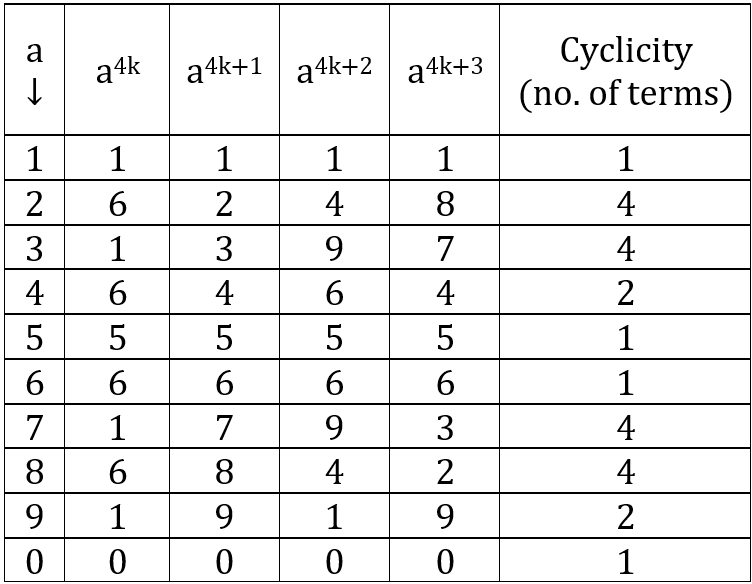# Concept: Unit's Digit

CONTENTS

INTRODUCTION

Remember:
• Units's digit of sum of two numbers same as unit's digit of sum of their respective unit's digits.
• Example: Units digit of 126 + 589 = Units digit of 6 + 9 = 5

• Units's digit of product of two numbers same as unit's digit of product of their respective unit's digits.
• Example: Units digit of 126 × 589 = Units digit of 6 × 9 = 4

CYCLICITY OF UNIT'S OF POWERS

Let us calculate the unit's digit of various powers of 2.

Unit's digit of 21 = 2
Unit's digit of 22 = 4
Unit's digit of 23 = 8
Unit's digit of 24 = 6
Unit's digit of 25 = 2
Unit's digit of 26 = 4
Unit's digit of 27 = 6
Unit's digit of 28 = 8
Unit's digit of 29 = 2
...

∴ We see that the unit's digit of powers of 2 repeates after every 4 powers. Hence, we can say that unit's digit of powers of 2 has a cyclicity of 4 terms.

Unit's digit of 24k = 6
Unit's digit of 24k+1 = 2
Unit's digit of 24k+2 = 4
Unit's digit of 24k+3 = 8

Remember:

Unit's digit of powers of:

• 2 has a cyclicity of 4 terms.
• 3 has a cyclicity of 4 terms.
• 4 has a cyclicity of 2 terms.
• 5 has a cyclicity of 1 term.
• 6 has a cyclicity of 1 term.
• 7 has a cyclicity of 4 terms.
• 8 has a cyclicity of 4 terms.
• 9 has a cyclicity of 2 terms.
• 0 has a cyclicity of 1 term.
• In general, we can say unit's digit of power of any number has a cyclicity of 4.​​​​​​​

Remember:
• Unit's digit of
• 4odd number = 4
• 4even number = 6

• Unit's digit of
• 9odd number = 9
• 9even number = 1

• Unit's digit of a4k+n = Unit's digit of an
where a is any single digit number.

• Units's digit (...abc)x = Units's digit of cx.
Example: Units digit of 12654 = Unit's digit of 654 = 6

• Unit's digit of (5 × odd number) = 5

• Unit's digit of (5 × even number) = 0

Example: Find unit's digit of (123)23

Solution:
Unit's digit of (123)23 = Unit's digit of 323

= Unit's digit of 34×5 + 3

= Unit's digit of 33

= 7

Example: Find unit's digit of (23454)769

Solution:
Unit's digit of (23454)769 = Unit's digit of 4769

We know Unit's digit of 4odd number = 4

∴ Unit's digit of (23454)769 = 4

Example: Find unit's digit of (582)945

Solution:
Unit's digit of (582)945 = Unit's digit of 2945

= Unit's digit of 24×236 + 1

= Unit's digit of 21

= 2

Example: Find unit's digit of ${\left(147\right)}^{{61}^{25}}$

Solution:
Unit's digit of ${\left(147\right)}^{{61}^{25}}$ = Unit's digit of ${\left(7\right)}^{{61}^{25}}$

Now, 6125 when divided by 4 leaves a remainder of 1 [Concept of Remainders]

∴ Unit's digit of ${\left(7\right)}^{{61}^{25}}$ = Unit's digit of 74k + 1

= Unit's digit of 71

= 7

## Feedback

Help us build a Free and Comprehensive CAT/MBA Preparation portal by providing us your valuable feedback about Apti4All and how it can be improved.